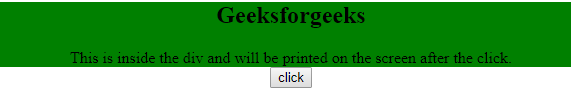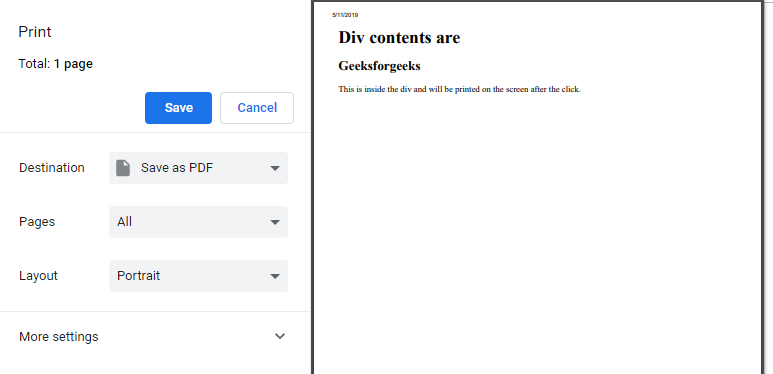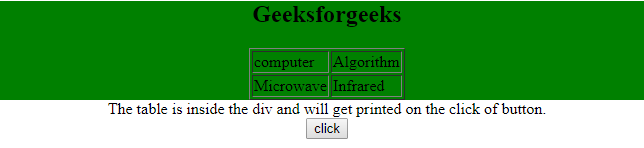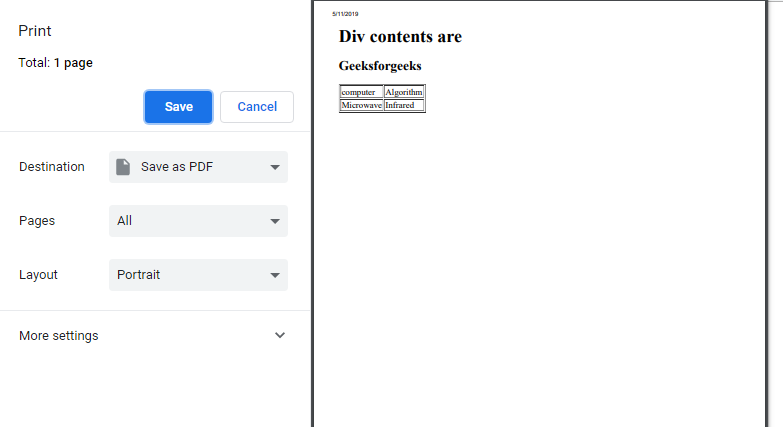# Print the content of a div element using JavaScript

• Difficulty Level : Basic
• Last Updated : 26 Jul, 2021

To print the content of div in JavaScript, first store the content of div in a JavaScript variable and then the print button is clicked. The contents of the HTML div element to be extracted. Then a JavaScript popup window is created and the extracted contents of the HTML div elements are written to the popup window and finally the window is printed using the JavaScript Window Print command.

Example 1: This example uses JavaScript window print command to print the content of div element.

Hey geek! The constant emerging technologies in the world of web development always keeps the excitement for this subject through the roof. But before you tackle the big projects, we suggest you start by learning the basics. Kickstart your web development journey by learning JS concepts with our JavaScript Course. Now at it's lowest price ever!

 ```<``html``>`` ` `<``head``>``    ``<``title``>``        ``Print the content of a div``    ````     ` `    ````    ``<``script``>``        ``function printDiv() {``            ``var divContents = document.getElementById("GFG").innerHTML;``            ``var a = window.open('', '', 'height=500, width=500');``            ``a.document.write('<``html``>');``            ``a.document.write('<``body` `> <``h1``>Div contents are <``br``>');``            ``a.document.write(divContents);``            ``a.document.write('');``            ``a.document.close();``            ``a.print();``        ``}``    `````` ` `<``body` `style``=``"text-align:center;"``>``     ` `    ``<``div` `id``=``"GFG"` `style``=``"background-color: green;"``>``         ` `        ``<``h2``>Geeksforgeeks``         ` `        ``<``p``>``            ``This is inside the div and will be printed``            ``on the screen after the click.``        ````    ````     ` `    ``<``input` `type``=``"button"` `value``=``"click"` `onclick``=``"printDiv()"``> ```` ` `                    `

Output:

• Before Clicking the button:• After Clicking the button:Example 2: This example uses JavaScript window print command to print the content of div element.

 ```<``html``>`` ` `<``head``>``    ``<``title``>``        ``Print the content of a div``    ````     ` `    ````    ``<``script``>``        ``function printDiv() {``            ``var divContents = document.getElementById("GFG").innerHTML;``            ``var a = window.open('', '', 'height=500, width=500');``            ``a.document.write('<``html``>');``            ``a.document.write('<``body` `> <``h1``>Div contents are <``br``>');``            ``a.document.write(divContents);``            ``a.document.write('');``            ``a.document.close();``            ``a.print();``        ``}``    `````` ` `<``body``>``    ``<``center``>``        ``<``div` `id``=``"GFG"` `style``=``"background-color: green;"``>``             ` `            ``<``h2``>Geeksforgeeks``             ` `            ``<``table` `border``=``"1px"``>``                ``<``tr``>``                    ``<``td``>computer``                    ``<``td``>Algorithm``                ````                ``<``tr``>``                    ``<``td``>Microwave``                    ``<``td``>Infrared``                ````            ````        ````         ` `        ``<``p``>``            ``The table is inside the div and will get``            ``printed on the click of button.``        ````         ` `        ``<``input` `type``=``"button"` `value``=``"click"``                    ``onclick``=``"printDiv()"``> ``    `` ```` ` `                                    `

Output:

• Before Clicking the button:• After Clicking the button:JavaScript is best known for web page development but it is also used in a variety of non-browser environments. You can learn JavaScript from the ground up by following this JavaScript Tutorial and JavaScript Examples.

My Personal Notes arrow_drop_up